# Strong tensor correlation in light nuclei with tensoroptimized

• Slides: 28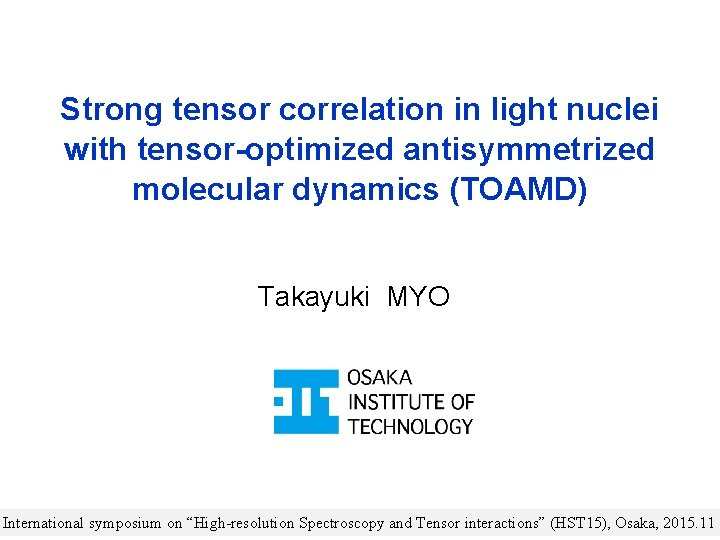Strong tensor correlation in light nuclei with tensor-optimized antisymmetrized molecular dynamics (TOAMD) Takayuki MYO International symposium on “High-resolution Spectroscopy and Tensor interactions” (HST 15), Osaka, 2015. 11Outline • Tensor Optimized Shell Model (TOSM) • Importance of 2 p 2 h excitation involving highmomentum component for tensor correlation • Applications to He, Li, Be isotopes • Tensor Optimized AMD (TOAMD) • Toward clustering with tensor correlation • Formulation given in PTEP 2015, 073 D 02 2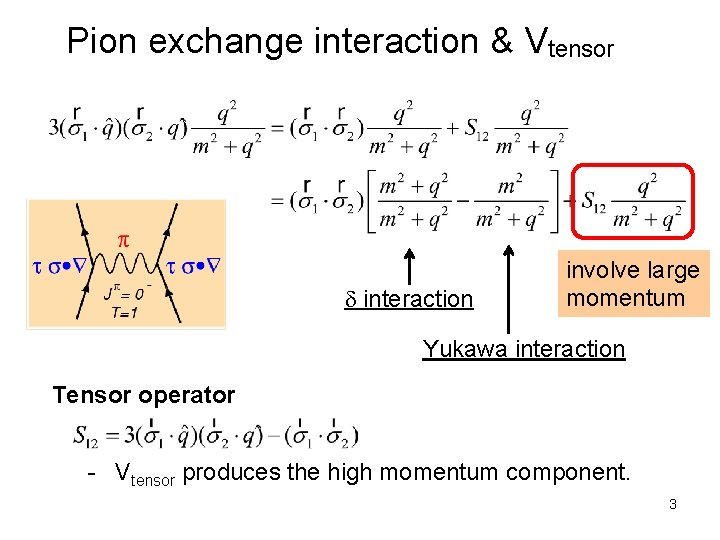Pion exchange interaction & Vtensor d interaction involve large momentum Yukawa interaction Tensor operator - Vtensor produces the high momentum component. 3Deuteron properties & tensor force Energy -2. 24 Me. V S Kinetic 19. 88 Central -4. 46 D Tensor -16. 64 LS -1. 02 P(L=2) 5. 77% AV 8’ Vcentral Vtensor r Rm(s)=2. 00 fm Rm(d)=1. 22 fm Radius 1. 96 fm d-wave is “spatially compact” (high momentum)Tensor-optimized shell model (TOSM) TM, Sugimoto, Kato, Toki, Ikeda PTP 117(2007)257 Shell model type configuration with mass number A (0 p 0 h+1 p 1 h+2 p 2 h) particle state Gaussian expansion for each orbit Gaussian basis function Hiyama, Kino, Kamimura PPNP 51(2003)223 c. m. excitation is excluded by Lawson’s method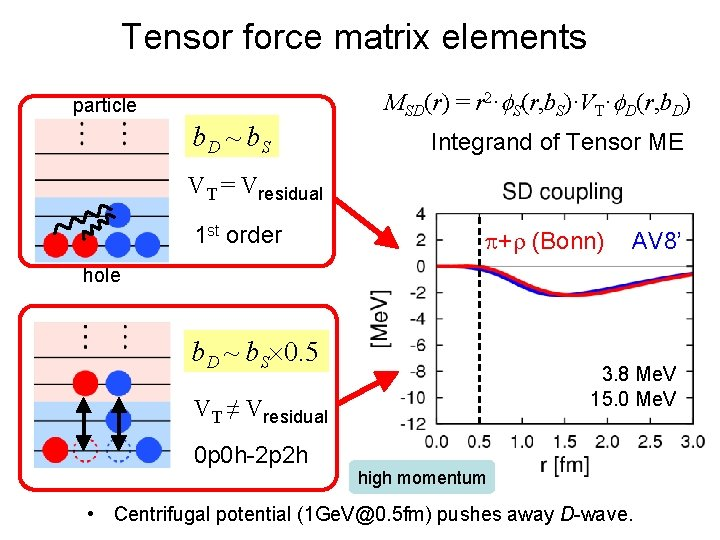Tensor force matrix elements MSD(r) = r 2·f. S(r, b. S)·VT·f. D(r, b. D) particle b. D ~ b. S Integrand of Tensor ME VT = Vresidual 1 st order p+r (Bonn) AV 8’ hole b. D ~ b. S 0. 5 3. 8 Me. V 15. 0 Me. V VT ≠ Vresidual 0 p 0 h-2 p 2 h high momentum • Centrifugal potential (1 Ge. [email protected] 5 fm) pushes away D-wave. 6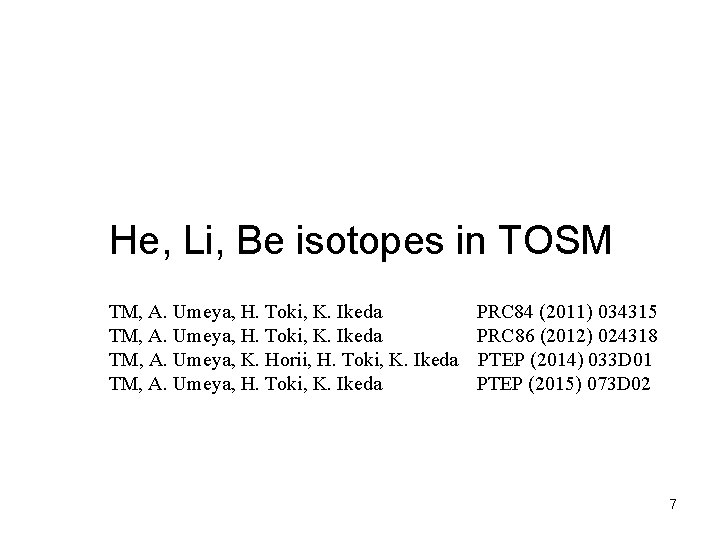He, Li, Be isotopes in TOSM TM, A. Umeya, H. Toki, K. Ikeda PRC 84 (2011) 034315 TM, A. Umeya, H. Toki, K. Ikeda PRC 86 (2012) 024318 TM, A. Umeya, K. Horii, H. Toki, K. Ikeda PTEP (2014) 033 D 01 TM, A. Umeya, H. Toki, K. Ikeda PTEP (2015) 073 D 02 7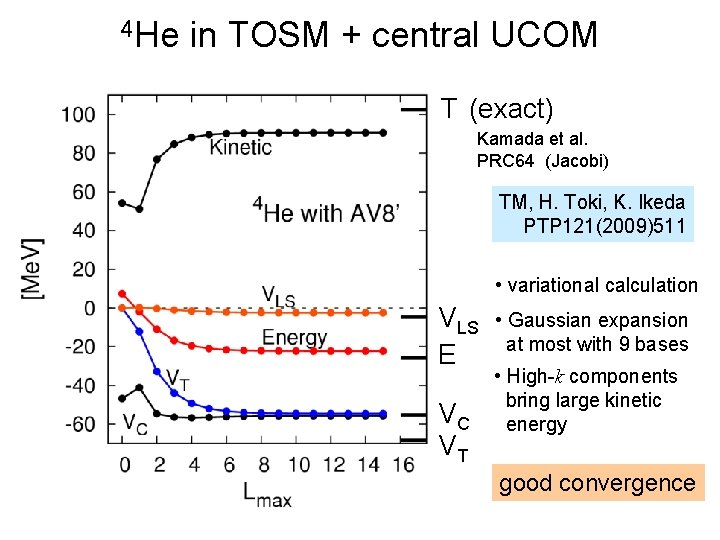4 He in TOSM + central UCOM T (exact) Kamada et al. PRC 64 (Jacobi) TM, H. Toki, K. Ikeda PTP 121(2009)511 • variational calculation VLS E VC VT • Gaussian expansion at most with 9 bases • High-k components bring large kinetic energy good convergence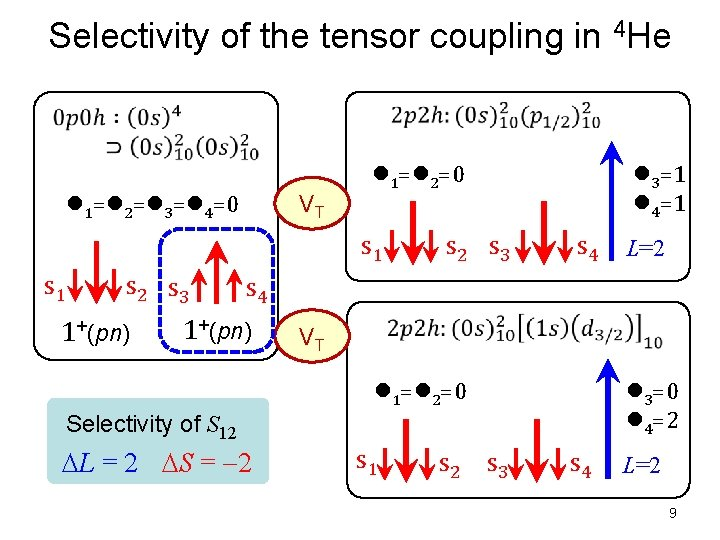Selectivity of the tensor coupling in 4 He l 1=l 2=l 3=l 4=0 VT s 1 s 2 s 3 1+(pn) l 3=1 l 4=1 l 1=l 2=0 s 2 s 3 s 4 L=2 s 4 1+(pn) VT l 1=l 2=0 l 3=0 l 4=2 Selectivity of S 12 DL = 2 DS = 2 s 1 s 2 s 3 s 4 L=2 95 -8 He with TOSM+UCOM • Excitation energies in Me. V TM, A. Umeya, H. Toki, K. Ikeda PRC 84 (2011) 034315 • Excitation energy spectra are reproduced well5 -9 Li with TOSM+UCOM • Excitation energies in Me. V TM, A. Umeya, H. Toki, K. Ikeda PRC 86(2012) 024318 • Excitation energy spectra are reproduced wellRadius of He & Li isotopes Expt TOSM with AV 8’ Halo Skin I. Tanihata et al. , PLB 289(‘ 92)261 O. A. Kiselev et al. , EPJA 25, Suppl. 1(‘ 05)215. A. Dobrovolsky, NPA 766(2006)1 G. D. Alkhazov et al. , PRL 78(‘ 97)231312 P. Mueller et al. , PRL 99(2007)252501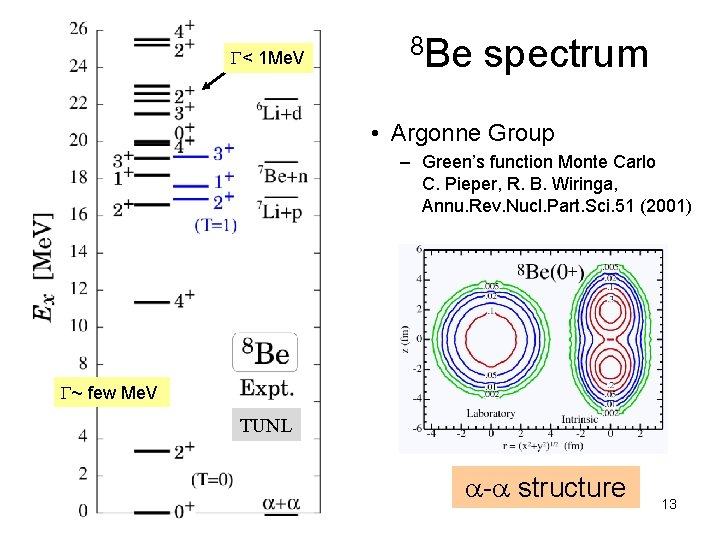G< 1 Me. V 8 Be spectrum • Argonne Group – Green’s function Monte Carlo C. Pieper, R. B. Wiringa, Annu. Rev. Nucl. Part. Sci. 51 (2001) G~ few Me. V TUNL a-a structure 138 Be in TOSM AV 8’ • VT 1. 1, VLS 1. 4 – simulate 4 He benchmark (Kamada et al. , PRC 64) • correct level order (T=0, 1) • tensor contribution : T=0 > T=1 • a : 0 p 0 h+2 p 2 h with high-k – 2 a needs 4 p 4 h. – spatial asymptotic form of 2 a Expt. (TUNL) TOSM a a clustering 14 TOAMDTensor-Optimized Antisymmetrized Molecular Dynamics (TOAMD) TM, H. Toki, K. Ikeda, H. Horiuchi, T. Suhara, PTEP 2015, 073 D 02 Toward the clustering with tensor correlation explicitly 15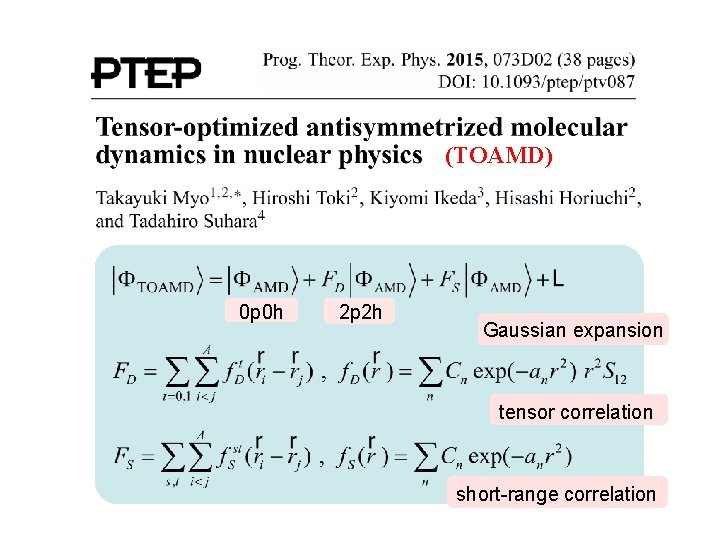(TOAMD) 0 p 0 h 2 p 2 h Gaussian expansion tensor correlation short-range correlationFormulation of TOAMD 0 p 0 h 2 p 2 h 4 p 4 h • 17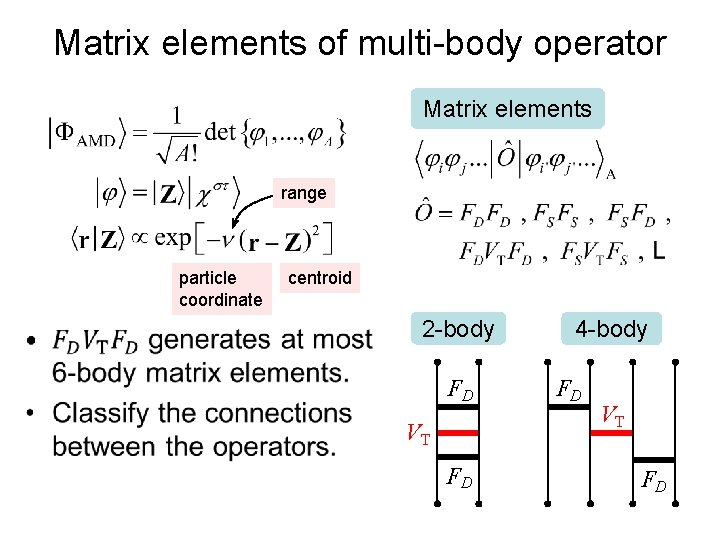Matrix elements of multi-body operator Matrix elements range particle coordinate • centroid 2 -body FD VT FD 4 -body FD VT FD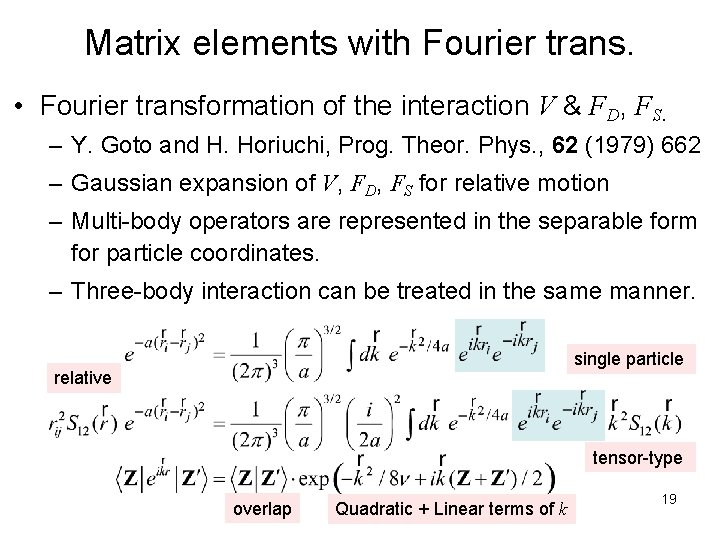Matrix elements with Fourier trans. • Fourier transformation of the interaction V & FD, FS. – Y. Goto and H. Horiuchi, Prog. Theor. Phys. , 62 (1979) 662 – Gaussian expansion of V, FD, FS for relative motion – Multi-body operators are represented in the separable form for particle coordinates. – Three-body interaction can be treated in the same manner. single particle relative tensor-type overlap Quadratic + Linear terms of k 19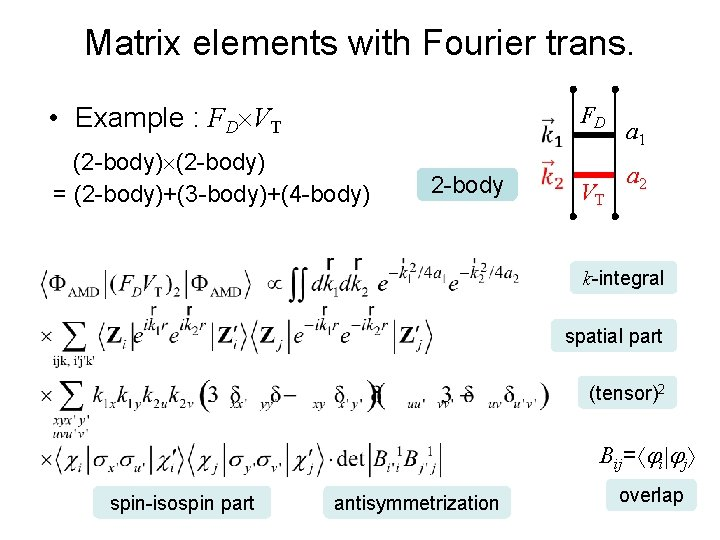Matrix elements with Fourier trans. • Example : FD VT (2 -body) = (2 -body)+(3 -body)+(4 -body) 2 -body FD VT a 1 a 2 k-integral spatial part (tensor)2 Bij= i j spin-isospin part antisymmetrization overlap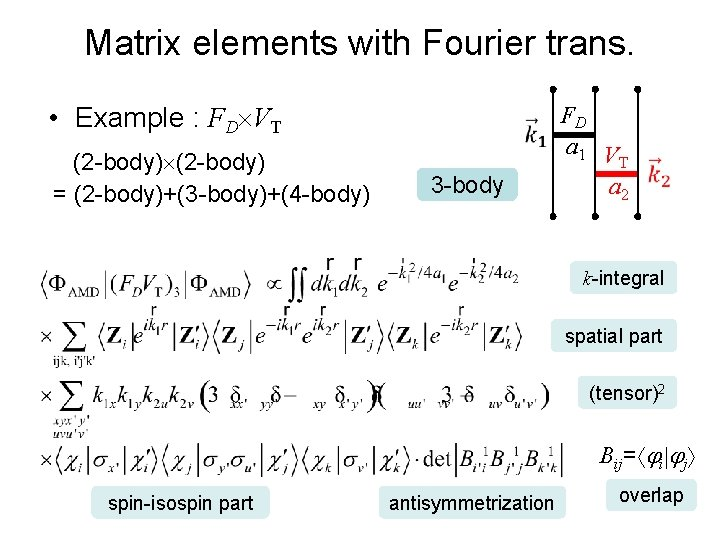Matrix elements with Fourier trans. • Example : FD VT (2 -body) = (2 -body)+(3 -body)+(4 -body) 3 -body FD a 1 V T a 2 k-integral spatial part (tensor)2 Bij= i j spin-isospin part antisymmetrization overlap 21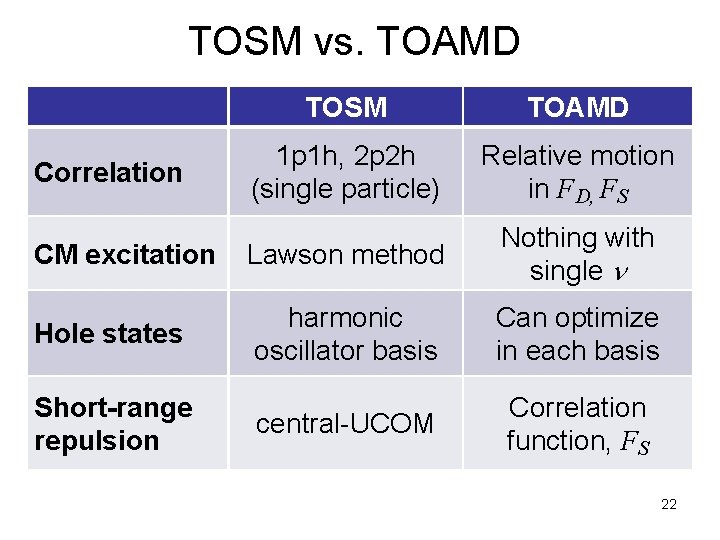TOSM vs. TOAMD TOSM TOAMD 1 p 1 h, 2 p 2 h (single particle) Relative motion in FD, FS CM excitation Lawson method Nothing with single n Hole states harmonic oscillator basis Can optimize in each basis central-UCOM Correlation function, FS Correlation Short-range repulsion 22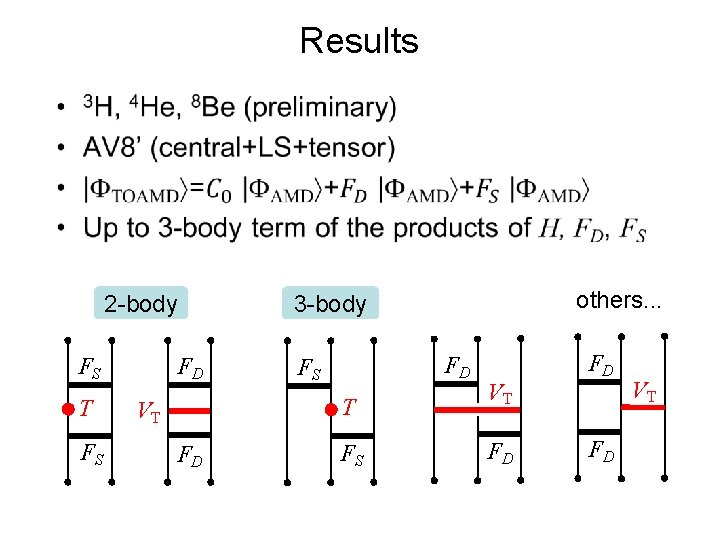Results • 2 -body FS T FS FD FD FS T VT FD others. . . 3 -body FS VT FD FD FD VT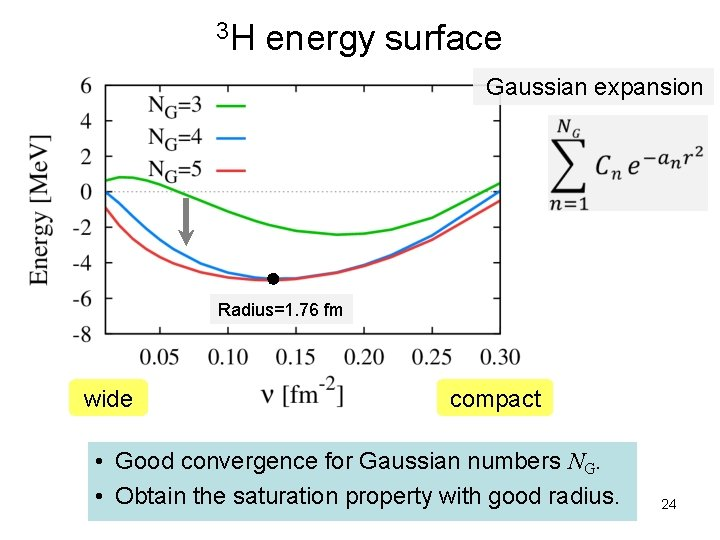3 H energy surface Gaussian expansion Radius=1. 76 fm wide compact • Good convergence for Gaussian numbers NG. • Obtain the saturation property with good radius. 24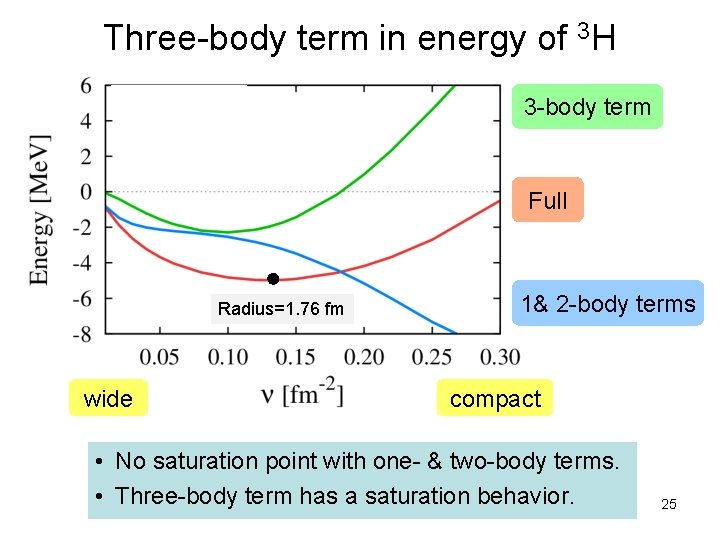Three-body term in energy of 3 H 3 -body term Full Radius=1. 76 fm wide 1& 2 -body terms compact • No saturation point with one- & two-body terms. • Three-body term has a saturation behavior. 25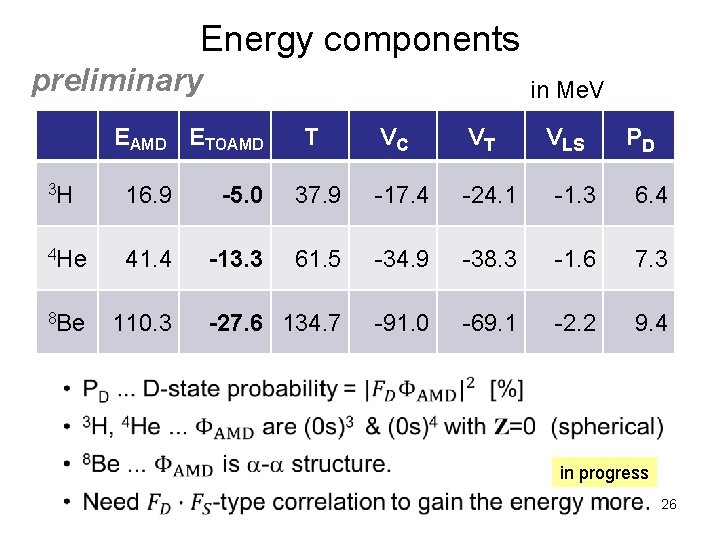Energy components preliminary in Me. V EAMD ETOAMD T VC VT VLS PD 3 H 16. 9 -5. 0 37. 9 -17. 4 -24. 1 -1. 3 6. 4 4 He 41. 4 -13. 3 61. 5 -34. 9 -38. 3 -1. 6 7. 3 8 Be 110. 3 -27. 6 134. 7 -91. 0 -69. 1 -2. 2 9. 4 • in progress 26Correlation functions FD, FS in 3 H FS 3 E 2 r 2 FD 3 E preliminary FS 1 E same trend in 3 H, 4 He, 8 Be • 27Summary • Tensor-Optimized Shell Model (TOSM) using Vbare. – Strong tensor correlation from 0 p 0 h-2 p 2 h involving high-k components. – He, Li, Be isotopes : Energy spectra, Radius of n-rich nuclei. – For 8 Be, two aspects : grand state region & highly excited states, which indicates of more configurations, such as a-a states. • Tensor-Optimized AMD (TOAMD). – Two-kinds of correlation functions FD (tensor) & FS (short-range) – Three-body term contributes to the energy saturation. – Ranges of FD , FS are not short. – Spatially compact behavior of FD produces high-k component. 28